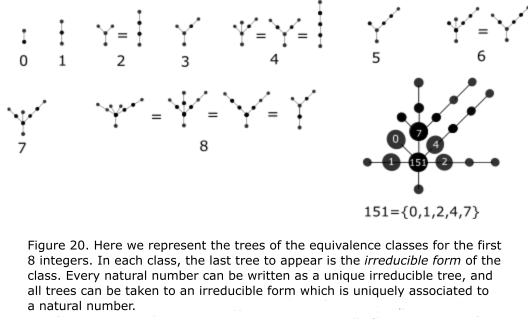Preprint Article Version 2 Preserved in Portico This version is not peer-reviewed

# A New Set Theory for Analysis

Version 1 : Received: 8 January 2019 / Approved: 9 January 2019 / Online: 9 January 2019 (15:48:12 CET)
Version 2 : Received: 9 January 2019 / Approved: 11 January 2019 / Online: 11 January 2019 (14:30:13 CET)

A peer-reviewed article of this Preprint also exists.

Ramírez, J.P. A New Set Theory for Analysis. Axioms 2019, 8, 31. Ramírez, J.P. A New Set Theory for Analysis. Axioms 2019, 8, 31.

Journal reference: Axioms 2019, 8, 31
DOI: 10.3390/axioms8010031

## Abstract

We present the real number system as a natural generalization of the natural numbers. First, we prove the co-finite topology, $Cof(\mathbb N)$, is isomorphic to the natural numbers. Then, we generalize these results to describe the continuum $[0,1]$. Then we prove the power set $2^{\mathbb Z}$ contains a subset isomorphic to the non-negative real numbers, with all its defining structure of operations and order. Finally, we provide two different constructions of the entire real number line. We see that the power set $2^{\mathbb N}$ can be given the defining structure of $\mathbb R$. The constructions here provided give simple rules for calculating addition, multiplication, subtraction, division, powers and rational powers of real numbers, and logarithms. The supremum and infimum are explicitly constructed by means of a well defined algorithm that ends in denumerable steps. In section 5 we give evidence our construction of $\mathbb N$ and $\mathbb R$ are canonical; these constructions are as natural as possible. In the same section, we propose a new axiomatic basis for analysis. In the last section we provide a series of graphic representations and physical models that can be used to represent the real number system. We conclude that the system of real numbers is completely defined by the order structure of $\mathbb N$.}## Keywords

General Topology, Axiomatic Set Theory, Real Analysis, Continuum, Graph Theory, Benacerraf's Identification Problem, Mathematical Structuralism

## Subject

MATHEMATICS & COMPUTER SCIENCE, Analysis

Views 0